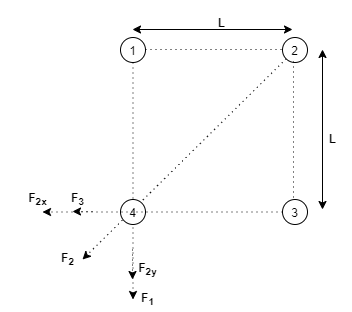# Problem: Four identical charges Q are placed at the corners of a square of side L.Find the magnitude total force exerted on one charge by the other three charges.

###### FREE Expert Solution

Coulomb's law:

$\overline{){\mathbf{F}}{\mathbf{=}}\frac{\mathbf{k}{\mathbf{q}}_{\mathbf{1}}{\mathbf{q}}_{\mathbf{2}}}{{\mathbf{r}}^{\mathbf{2}}}}$, where k is Coulomb constant and r is the separation between q1 and q2.

Let's visualize this problem in a diagram:Let the forces be acting on the charge at position 4.

F3 = - kQ2/L2

The x-component of the electric force on charge 4 due to charge 2 is given by:

79% (199 ratings)###### Problem Details

Four identical charges Q are placed at the corners of a square of side L.

Find the magnitude total force exerted on one charge by the other three charges.

Frequently Asked Questions

What scientific concept do you need to know in order to solve this problem?

Our tutors have indicated that to solve this problem you will need to apply the Coulomb's Law (Electric Force) concept. You can view video lessons to learn Coulomb's Law (Electric Force). Or if you need more Coulomb's Law (Electric Force) practice, you can also practice Coulomb's Law (Electric Force) practice problems.